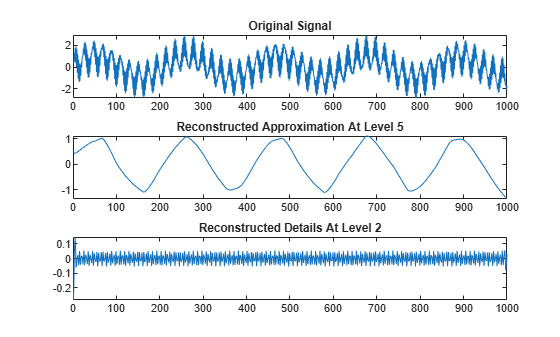# wrcoef

Reconstruct single branch from 1-D wavelet coefficients

## Syntax

``x = wrcoef(type,c,l,wname)``
``x = wrcoef(type,c,l,LoR,HiR)``
``x = wrcoef(___,n)``

## Description

````x = wrcoef(type,c,l,wname)` reconstructs the coefficients vector of type `type` based on the wavelet decomposition structure `[c,l]` of a 1-D signal (see `wavedec` for more information) using the wavelet specified by `wname`. The coefficients at the maximum decomposition level are reconstructed. The length of `x` is equal to the length of the original 1-D signal.```
````x = wrcoef(type,c,l,LoR,HiR)` uses the reconstruction filters `LoR` and `HiR`.```

example

````x = wrcoef(___,n)` reconstructs the coefficients at level `n` using any of the previous syntaxes.```

## Examples

collapse all

```load sumsin s = sumsin;```

Perform a level 5 wavelet decomposition of the signal using the `sym4` wavelet.

`[c,l] = wavedec(s,5,'sym4');`

Reconstruct the approximation coefficients at level 5 from the wavelet decomposition structure `[c,l]`.

`a5 = wrcoef('a',c,l,'sym4');`

Reconstruct the detail coefficients at level 2.

`d2 = wrcoef('d',c,l,'sym4',2);`

Plot the original signal and reconstructed coefficients.

```subplot(3,1,1) plot(s) title('Original Signal') subplot(3,1,2) plot(a5) title('Reconstructed Approximation At Level 5') subplot(3,1,3) plot(d2) title('Reconstructed Details At Level 2')```## Input Arguments

collapse all

Coefficients to reconstruct, specified as `'a'` or `'d'`, for approximation or detail coefficients, respectively.

Wavelet decomposition of a 1-D signal, specified as a real-valued vector. The vector contains the wavelet coefficients. The bookkeeping vector `l` contains the coefficients by level. See `wavedec`.

Data Types: `double` | `single`

Bookkeeping vector, specified as a vector of positive integers. The bookkeeping vector is used to parse the coefficients in the wavelet decomposition `c` by level. See `wavedec`.

Data Types: `double` | `single`

Analyzing wavelet used to create the wavelet decomposition structure `[c,l]`, specified as a character vector or string scalar. `wrcoef` supports only orthogonal or biorthogonal wavelets. See `wfilters`.

Wavelet reconstruction filters, specified as a pair of even-length real-valued vectors. `LoR` is the lowpass reconstruction filter, and `HiR` is the highpass reconstruction filter. The lengths of `LoR` and `HiR` must be equal. See `wfilters` for additional information.

Coefficients level, specified as a nonnegative integer. When `type` is `'a'`, `n` is allowed to be 0. Otherwise, `n` is a strictly positive integer such that `n ≤ length(l)-2`. The default value of `n` is `length(l)-2`.

## Version History

Introduced before R2006a